## Chapter 5. Area operations

If thence he 'scappe, into whatever world, Or unknown region.

--Milton

In Chapter 4, Point operations we looked at operations that calculated the new sample values of a pixel based on the value of the original pixel alone. In this chapter we look at the extension of that concept, operations that take their input from a local neighbourhood of the original pixel.

## Sampling area

We can sample with different input windows the most common are a square or circle centered on the pixel as shown in Figure 5.1, “Sliding window”.

Figure 5.1. Sliding window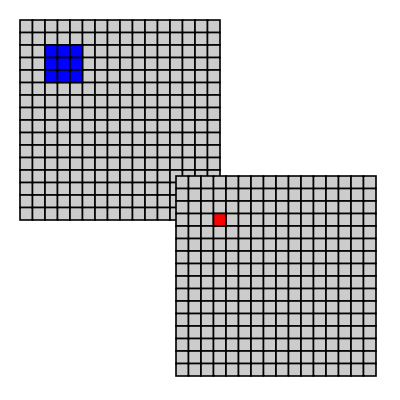The 3×3 blue area in the raster on the left, indicates which pixels contribute to the single red pixel in the destination image.

### Edge handling

When sampling a local area around a pixel, we need to decide what to do when part of the sampling window is outside the source image. The common way to provide a "fake" value for data outside the image are:

• Make them transparent.
• Give them a color (for instance black).
• Nearest valid pixel.
• Mirror the image over the edge.
• Wrap to other side of image.

In gluas the global variable edge_duplicate can be set to 1, this makes gluas return the nearest valid pixel instead of transparent/black.

Figure 5.2. Over the edge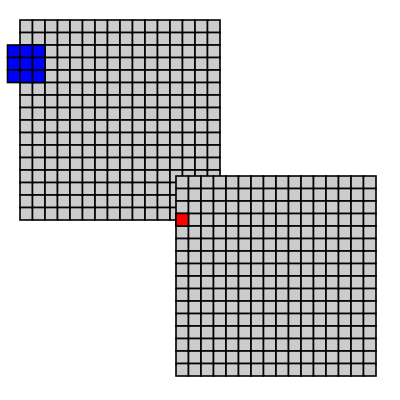The 3×3 blue area in the raster on the left, indicates which pixels contribute to the single red pixel in the destination image.

## Convolution Matrix

A special group of operations are the kernel filters, which use a table of numbers (a matrix) as input. The matrix gives us the weight to be given each input sample. The matrix for a kernel filter is always square and the number of rows/columns are odd. Many image filters can be expressed as convolution filters some are listed in the sourcelisting in Figure 5.3, “convolve”.

Each sample in the sampling window is weighted with the corresponding weight in the convolution matrix. The resulting sum is divided by the divisor (for most operations this is assumed to be the sum of the weights in the matrix). And finally an optional offset might be added to the result.

Figure 5.3. convolve```-- 3x3 convolution filter

edge_duplicate=1;

function convolve_get_value (x,y, kernel, divisor, offset)
local i, j, sum
sum_r = 0
sum_g = 0
sum_b = 0

for i=-1,1 do
for j=-1,1 do
r,g,b = get_rgb (x+i, y+j)
sum_r = sum_r + kernel[j+2][i+2] * r
sum_g = sum_g + kernel[j+2][i+2] * g
sum_b = sum_b + kernel[j+2][i+2] * b
end
end
return sum_r/divisor + offset, sum_g/divisor+offset, sum_b/divisor+offset
end

function convolve_value(kernel, divisor, offset)
for y=0, height-1 do
for x=0, width-1 do
r,g,b = convolve_get_value (x,y, kernel, divisor, offset)
set_rgb (x,y, r,g,b)
end
progress (y/height)
end
flush ()
end

function emboss()
kernel = { { -2, -1,  0},
{ -1,  1,  1},
{  0,  1,  2}}
divisor = 1
offset  = 0
convolve_value(kernel, divisor, offset)
end

function sharpen()
kernel = { { -1, -1, -1},
{ -1,  9, -1},
{ -1, -1, -1}}
convolve_value(kernel, 1, 0)
end

function sobel_emboss()
kernel = { { -1, -2, -1},
{  0,  0,  0},
{  1,  2,  1}}
convolve_value(kernel, 1, 0.5)
end

function box_blur()
kernel = { {  1,  1,  1},
{  1,  1,  1},
{  1,  1,  1}}
convolve_value(kernel, 9, 0)
end

sharpen ()
```

## Smoothing filters

### Box blur

A convolution matrix with all weights set to 1.0 is a blur, blurring is an operation that is often performed and can be implemented programmatically faster than the general convolution. A blur can be seen as the average of an area, or a resampling which we'll return to in a later chapter.

Figure 5.4. blur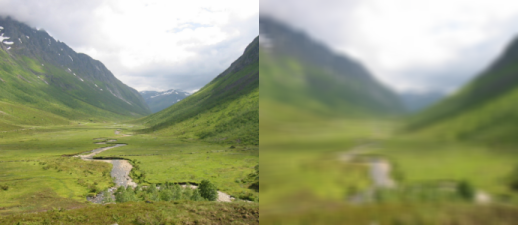```-- a box blur filter implemented in lua

edge_duplicate = 1;

local x,y
local rsum,gsum,bsum,asum=0,0,0,0
local count=0

local r,g,b,a= get_rgba(x,y)

rsum, gsum, bsum, asum = rsum+r, gsum+g, bsum+b, asum+a

count=count+1
end
end
return rsum/count, gsum/count, bsum/count, asum/count
end

local x,y

for y = 0, height-1 do
for x = 0, width-1 do
end
progress (y/height)
end
flush ()
end

-- seperation into two passes, greatly increases the speed of this
-- filter

local x,y
local rsum,gsum,bsum,asum=0,0,0,0
local count=0

y=sample_y
local r,g,b,a= get_rgba(x,y)

rsum, gsum, bsum, asum = rsum+r, gsum+g, bsum+b, asum+a

count=count+1
end
return rsum/count, gsum/count, bsum/count, asum/count
end

for y = 0, height-1 do
for x = 0, width-1 do
end
progress(y/height)
end
flush()
end

local x,y
local rsum,gsum,bsum,asum=0,0,0,0
local count=0

x=sample_x
local r,g,b,a= get_rgba(x,y)

rsum, gsum, bsum, asum = rsum+r, gsum+g, bsum+b, asum+a

count=count+1
end
return rsum/count, gsum/count, bsum/count, asum/count
end

for y = 0, height-1 do
for x = 0, width-1 do
end
progress(y/height)
end
flush()
end

end

blur_fast(4)
```

### Gaussian blur

A better looking blur is achieved by setting the weights to a gaussian distribution around the active pixel.

A gaussian blur works by weighting the input pixels near the center of ther sampling window higher than the input pixels further away.

One way to approximate a small gaussian blur, is to set the center pixel in a 3×3 matrix to 2, while the surrounding are set to 1, and set the weight to 10.

### Speeding up blur by decomposing

A blur is a time consuming operation especially if the radius is large. A way to optimize a blur is to observe that first blurring horizontally and then vertically gives the same result as doing both at the same time. This has been done to the blur_fast in Figure 5.4, “blur” and can also be done for gaussian blurs.

### Edge preserving

Edge preserving smoothing filters are useful for automated feature detection or even just for artistic effects.

#### Kuwahara

The kuwahara filter is an edge preserving blur filter. It works by calculating the mean and variance for four subquadrants, and chooses the mean value for the region with the smallest variance.

Figure 5.5. kuwahara```-- Kuwahara filter
--
--
-- Performs the Kuwahara Filter. This filter is an edge-preserving filter.
--
--
-- ( a  a  ab   b  b)
-- ( a  a  ab   b  b)
-- (ac ac abcd bd bd)
-- ( c  c  cd   d  d)
-- ( c  c  cd   d  d)
--
-- In each of the four regions (a, b, c, d), the mean brightness and the variance are calculated. The
-- output value of the center pixel (abcd) in the window is the mean value of that region that has the
-- smallest variance.
--
-- description copied from http://www.incx.nec.co.jp/imap-vision/library/wouter/kuwahara.html
--
-- implemented by Øyvind Kolås <oeyvindk@hig.no> 2004

-- the sampling window is:  width=size*2+1  height=size*2+1

size = 4

edge_duplicate = 1;

-- local function to get the mean value, and
-- variance from the rectangular area specified
function mean_and_variance (x0,y0,x1,y1)
local variance
local mean

local min         = 1.0
local max         = 0.0
local accumulated = 0
local count       = 0

local x, y

for y=y0,y1 do
for x=x0,x1 do
local v = get_value(x,y)

accumulated   = accumulated + v
count         = count + 1

if v<min then min = v end
if v>max then max = v end

end
end

variance = max-min
mean     = accumulated /count

return mean, variance
end

-- local function to get the mean value, and
-- variance from the rectangular area specified
function rgb_mean_and_variance (x0,y0,x1,y1)
local variance
local mean
local r_mean
local g_mean
local b_mean

local min           = 1.0
local max           = 0.0
local accumulated_r = 0
local accumulated_g = 0
local accumulated_b = 0
local count         = 0

local x, y

for y=y0,y1 do
for x=x0,x1 do
local v     = get_value(x,y)
local r,g,b = get_rgb (x,y)

accumulated_r   = accumulated_r + r
accumulated_g   = accumulated_g + g
accumulated_b   = accumulated_b + b
count         = count + 1

if v<min then min = v end
if v>max then max = v end

end
end

variance = max-min
mean_r   = accumulated_r /count
mean_g   = accumulated_g /count
mean_b   = accumulated_b /count

return mean_r, mean_g, mean_b, variance
end

-- return the kuwahara computed value
function kuwahara(x, y, size)
local best_mean     = 1.0
local best_variance = 1.0

local mean, variance

mean, variance = mean_and_variance (x-size, y-size, x, y)

if variance < best_variance then
best_mean     = mean
best_variance = variance
end

mean, variance = mean_and_variance (x, y-size, x+size,y)

if variance < best_variance then
best_mean     = mean
best_variance = variance
end

mean, variance = mean_and_variance (x, y, x+size, y+size)

if variance < best_variance then
best_mean     = mean
best_variance = variance
end

mean, variance = mean_and_variance (x-size, y, x,y+size)

if variance < best_variance then
best_mean     = mean
best_variance = variance
end

return best_mean
end

-- return the kuwahara computed value
function rgb_kuwahara(x, y, size)
local best_r, best_g, best_b
local best_variance = 1.0

local r,g,b, variance

r,g,b, variance = rgb_mean_and_variance (x-size, y-size, x, y)

if variance < best_variance then
best_r, best_g, best_b = r, g, b

best_variance = variance
end

r,g,b, variance = rgb_mean_and_variance (x, y-size, x+size,y)

if variance < best_variance then
best_r, best_g, best_b = r, g, b
best_variance = variance
end

r,g,b, variance = rgb_mean_and_variance (x, y, x+size, y+size)

if variance < best_variance then
best_r, best_g, best_b = r, g, b
best_variance = variance
end

r,g,b, variance = rgb_mean_and_variance (x-size, y, x,y+size)

if variance < best_variance then
best_r, best_g, best_b = r, g, b
best_variance = variance
end

return best_r, best_g, best_b
end

for y=0, height-1 do
for x=0, width-1 do
set_rgb (x,y, r,g,b)
end
progress (y/height)
end
end

kuwahara(2)
```

#### Symmetric Nearest Neighbour

An improvement over the kuwahara filter is the symmetric nearest neighbour filter that compares symmetric pairs/quadruples of pixels with the center pixel and only takes into account the one from this set which is closest in value to the center pixel.

Figure 5.6. snn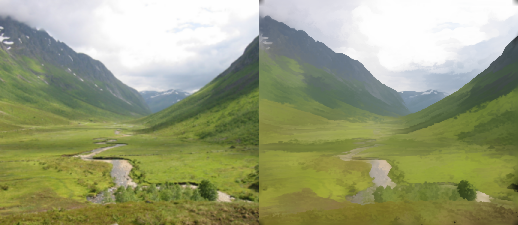```-- Symmetric Nearest Neighbour as described by Hardwood et al in Pattern
-- recognition letters 6 (1987) pp155-162.

local x,y
for y=0, height-1 do
for x=0, width-1 do
local sum=0.0
local count=0.0
local u,v

local valueC = get_value (x,y)

local valueA = get_value (x+u,y+v)
local valueB = get_value (x-u,y-v)

if (math.abs(valueC-valueA) <
math.abs(valueC-valueB)) then
sum = sum + valueA
else
sum = sum + valueB
end
count = count + 1
end
end
set_value (x,y, sum/count)
end
progress (y/height)
end
flush()
end

-- snn(6)

-- and a color version operating in CIE Lab

function deltaE(l1,a1,b1,l2,a2,b2)
return math.sqrt( (l1-l2)*(l1-l2) + (a1-a2)*(a1-a2) + (b1-b2)*(b1-b2))
end

local x,y
for y=0, height-1 do
for x=0, width-1 do
local sumL=0.0
local suma=0.0
local sumb=0.0
local count=0.0
local u,v

local lc,ac,bc = get_lab (x,y)

local l1,a1,b1 = get_lab (x+u,y+v)
local l2,a2,b2 = get_lab (x-u,y-v)

if (deltaE(lc,ac,bc,l1,a1,b1) <
deltaE(lc,ac,bc,l2,a2,b2)) then
sumL = sumL + l1
suma = suma + a1
sumb = sumb + b1
else
sumL = sumL + l2
suma = suma + a2
sumb = sumb + b2
end
count = count + 1
end
end
set_lab (x,y, sumL/count, suma/count, sumb/count)
end
progress (y/height)
end
flush()
end

snn_color(6)```

## Rank/statistical filters

Rank filters operate statistically on the neighbourhood of a pixel. The tree most common rank filters are median, minimum, maximum.

### Median

The median filter sorts the sample values in theneighbourhood, and then picks the middle value. The effect is that noise is removed while detail is kept. See also kuwahara. The median filter in The GIMP is called Despeckle .

Figure 5.7. median```-- a median rank filter

edge_duplicate = 1;

local x,y
local sample = {}
local samples = 0

local value = get_value(x,y)

sample[samples] = value
samples = samples + 1
end
end

table.sort (sample)
local mid = math.floor(samples/2)
if math.mod(samples,2) == 1 then
median = sample[mid+1]
else
median = (sample[mid]+sample[mid+1])/2
end

return median
end

local x,y

for y = 0, height-1 do
for x = 0, width-1 do
end
progress (y/height)
end
flush ()
end

median(3)
```

Unsharp mask is a quite novel way to sharpen an image, it has it's origins in darkroom work. And is a multistep process.

1. create a duplicate image

2. blur the duplicate image

3. calculate the difference between the blurred and the original image

4. add the difference to the original image

The unsharp mask process works by exaggerating the mach band effect .

## Misc area filters.

In this section some stranger filters are listed, these filters are not standard run of the mill image filters. But simple fun filters that fall into the category of area filters.

### Jitter

Pick an a random pixel within the sampling window.

Figure 5.8. jitter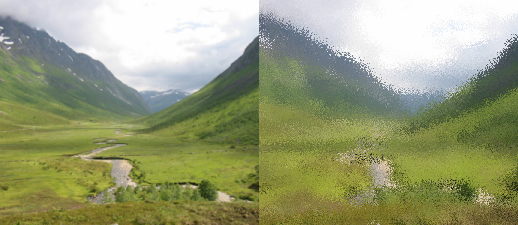```-- a jittering filter implemented in lua

edge_duplicate=1 -- set edge wrap behavior to use nearest valid sample

function jitter(amount)
local x,y
var2=var1
for y = 0, height-1 do
for x = 0, width-1 do
local nx,ny
local r,g,b,a
nx=x+(math.random()-0.5)*amount
ny=y+(math.random()-0.5)*amount
r,g,b,a=get_rgba(nx,ny)
set_rgba(x,y, r,g,b,a)
end
progress(y/height)
end
end

jitter(10)
```

## Excercises

### 1. Extend the convolve example

Extend the convolve example, making it possible for it to accept a 7×7 matrix for it's values, add automatic summing to find the divisor and experiment with various sampling shapes.